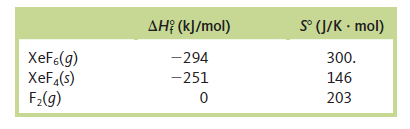# Problem: Given the thermodynamic data below, calculate ΔS and ΔS surr for the following reaction at 25°C and 1 atm:                       XeF  6(g) →XeF4(s) + F2(g)

⚠️Our tutors found the solution shown to be helpful for the problem you're searching for. We don't have the exact solution yet.

###### Problem Details

Given the thermodynamic data below, calculate ΔS and ΔS surr for the following reaction at 25°C and 1 atm:

XeF  6(g) →XeF4(s) + F2(g)